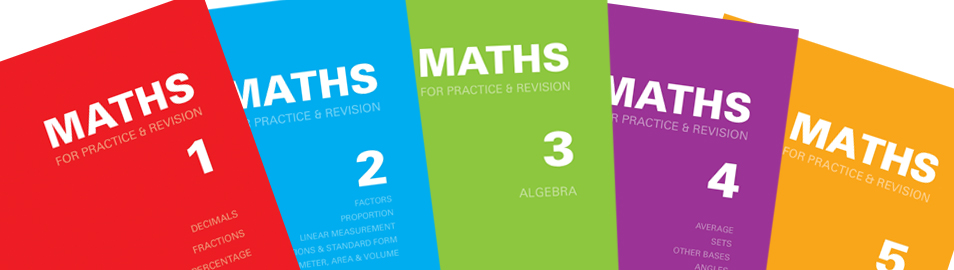This set of four books which are easy to use, provides an introduction to basic arithmetic, covering the four rules (addition, subtraction, multiplication and division) with integers, decimals and vulgar fractions.

The books are set out in a similar way to the 'senior' Maths for Practice & Revision series but they progress at a gentler pace. The text on each left-hand page explains the method(s) with worked examples, the corresponding right-hand page giving related questions for the student to try. At the end of each book there are fifty mixed questions of the types found on earlier pages.

A separate booklet gives answers to all the questions in the series.

Size of books 240 x 168 mm. Laminated card covers.# Book A

Price £4.95.

An introduction to counting, addition, subtraction and basic shapes (plane and solid).

Suitable for students aged 6 or over.

72 pages. Red cover.

ISBN 978-1-872686-24-0.

# Book B

Price £4.95.

Multiplication and division in simple stages, including squares, cubes, area of rectangle and very simple fractions.

Suitable for students aged 6 or over.

72 pages. Green cover.

ISBN 978-1-872686-26-4.

# Book C

Price £4.95.

First steps in decimals including column values, addition, subtraction, multiplication, division and money (coins, banknotes and calculations).

Suitable for students aged 7 or over.

64 pages. Blue cover.

ISBN 978-1-872686-27-1.

# Book D

Price £4.95.

An introduction to vulgar fractions.

Suitable for students aged 8 or over.

64 pages. Gold cover.

ISBN 978-1-872686-28-8.

Price £4.95.

Answers to all questions in Early Maths Books A, B, C and D.

48 pages. Pink card cover.